by
under ,
tagged ,

# Appendix of the Jeffreys’s paper

The following calculations are based on Jeffreys’s (1961, p. 269) Theory of Probability and elaborates why he choose to use a symmetric proper weighting function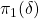on the test relevant parameter the population effect size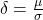.

First, to relate the observed-value to the population effect size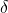within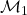, Jeffreys rewrote the likelihood of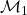in terms of the effect sizeand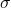. To calculate the weighted likelihood ofhe then choose to set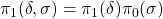. By assigning the same weighting function toas was done for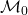, we obtain:

(1)The remaining task is to specify, the weighting function for the test-relevant parameter. Jeffreys proposed his weighting functionbased on desiderata obtained from hypothetical, extreme data.

### Predictive matching: SymmetricThe first “extreme” case Jeffreys discusses is when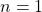; this automatically yieldsregardless of the value of. Jeffreys noted that a single datum cannot provide support for, as any deviation of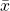from zero can also be attributed to our lack of knowledge of. Hence, nothing is learned from only one observation and consequently the Bayes factor should equal 1 whenever.

To ensure thatwhenever, Jeffreys (1961, p. 269) entered, thus,andinto Eq. 2 and noted thatequals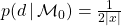, ifis taken to be symmetric around zero. The proof assumes thatand uses the transformation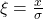, thus,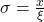and. Hence, by symmetry ofwe get

(2)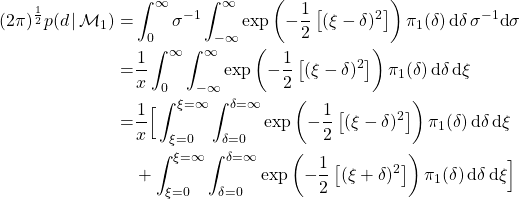By swapping the order of integration (Fubini) then yields an integral in terms of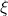that yields the normalisation constant of a normal distribution, that is,

(3)Hence, forsymmetric around zero the weighted likelihood of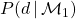is then equal to that of the null model wheneverand.